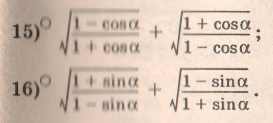# How do you simplify sqrt97?

How do you simplify sqrt97?

ReportAnswer 1

You can't, because it is a prime number. You can use a calculator to find it's approximately $9.85$.

##### Add Answer of: How do you simplify sqrt97?
Similar Homework Help Questions
• ### How do you simplify this

How do you simplify this? 1/{(x/y)+1}multipy numerator and denominator by y. y (1)/y(x/y +1) then simplifyy/1+x?

• ### How do you simplify this

How do you simplify this? --- --- / 1 / 5/ - * /18 / 3 /The hyphens and slashes are meant to be radical signs.Thank you!

• ### ____ How do you simplify these

____ How do you simplify these? // 24 Including numbers such as 40 60 and 48?

• ### How do you simplify?

sec^2(t)/(sec^2(t)-1) 

• ### How do you simplify it??• ### How do you simplify?

64x^3 +27factor

• ### How do you simplify this?

(sqrt48n^6)/(sqrt6n^3)

• ### How do you simplify this problem

How do you simplify this problem?(-3y)+(-2)+(-8x)+5y-(-8x)

Free Homework App